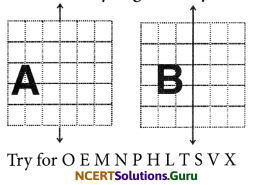# NCERT Solutions for Class 6 Maths Chapter 13 Symmetry Ex 13.3

These NCERT Solutions for Class 6 Maths Chapter 13 Symmetry Ex 13.3 Questions and Answers are prepared by our highly skilled subject experts.

## Exercise 13.3

Question 1.
Find the number of lines of symmetry in each of the following shapes. How will you check your answers?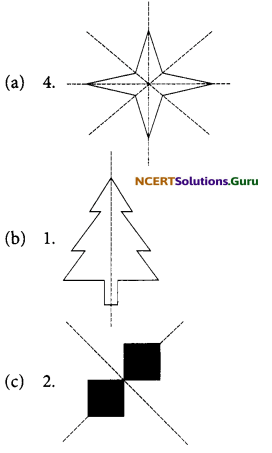Question 2.
Copy the following drawing on squared paper. Complete each one of them such that the resulting figure has two dotted lines as two lines of symmetry.
How did you go about completing the picture?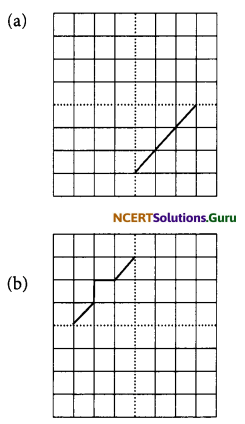Question 3.
How did you go about completing the picture?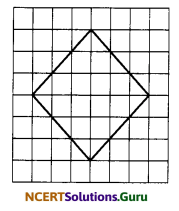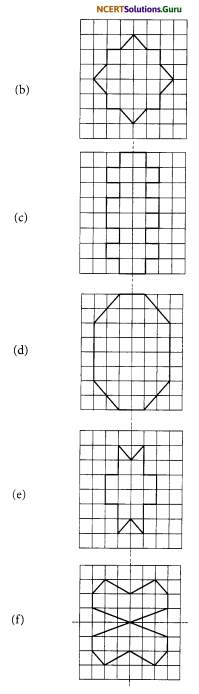Question 4.
In each figure below, a letter of alphabet is shown along with a vertical line. Take
the mirror image of the letter in the given line. Find which letters look the same after reflection (i.e., which letters look the same in the image) and which do not. Can you guess why?